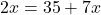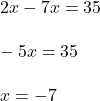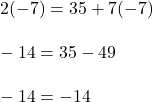## Twice a number is equal to 35more than 7 times the number .find the number

Question

Twice a number is equal to 35more than 7 times the number .find the number

in progress 0
7 months 2021-07-18T00:02:13+00:00 1 Answers 2 views 0

the number is -7

Step-by-step explanation:

so the equation looks like thistwice the number equals 35 more than 7 times the number

now solver for xand the number is -7